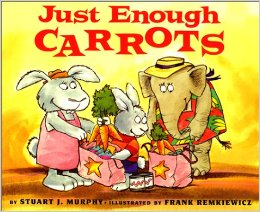# Greater Than

16 teachers like this lesson
Print Lesson

## Objective

SWBAT model and compare two digit numbers to determine which is greater.

#### Big Idea

Greater Gator! This lesson has students making models to compare two digit numbers.

## Activating Strategy

10 minutes

I like to start this lesson by reading the book Just Enough Carrots.  If the book isn't available, here is a link to the video on You Tube.I like to start off using this book because it goes through real life connections of using greater than and less than.  During the book, there are ample opportunities to discuss numbers that are greater than and less than.

This standard states that students need to be able to compare numbers based on the tens or ones place using <, >, and =. (1.NBT.B.3)  This lesson teaches the greater than portion of the standard.

## Teaching Strategies

15 minutes

Write the numbers 36 and 31 on the board.  Have a student come to the board and draw a picture to represent the tens and ones for 36 and 31.  Discuss how they both have the same number of tens (3) but the number of ones is different.  There are more ones in 36 than in 31. Talk about how 36 is greater than 31.

Ask the students what they know about the terms more and greater.  Guide the discussion:

• I have a bowl of 15 cubes and a bowl of 20 cubes.  Which bowl has more cubes? (20 cubes is more than 15 cubes.)
• Which number is greater, 15 or 20? (20 is greater than 15.)

Read the following problem aloud.  Have children use base ten blocks to model the two numbers.  Remind children that 1 ten is the same as 10 ones.

Which number is greater, 65 or 56?

• Both numbers have a 5 and a 6.  How are your models different?  (65 has 6 tens and 5 ones, and 56 has 5 tens and 6 ones.)
• How can we use the drawings to compare the numbers? (I can match the tens of each number to see which number has more tens.  The number with more tens is the greater number.)
• Do we need to compare the ones?  Explain.  (No, the amount of tens is different and that already tells me which number is greater.)

Write the numbers 52 and 17 on the board/chart paper.  Ask students to model using base ten blocks to make the two numbers.  Ask the students:

• Why do you first compare the tens? (If the tens are different, then the number with more tens is greater.  You don’t need to compare the ones.)

I have a student volunteer come to the board and model how to draw the two numbers to determine which number is greater.

Write the numbers 17 and 15 on the board/chart paper.  Have students model the numbers using base ten blocks.  Ask the students:

• Do we compare the tens?  Why or why not?  (No, they both have 1 ten.)
• Do we compare the ones?  Why or why not? (Yes, 17 has 7 ones and 15 has 5 ones.  17 is the greater number.)

Having students make models will  help them to transition from concrete to abstract ideas.  Modeling with math helps students to make connections with the different representations. (MP4)

## Independent Practice

30 minutes

For the independent portion of this lesson, I like to hand out this Greater Than_worksheet.docx.

Here is an explanation of why I like to use this worksheet for this lesson:

For those struggling students, encourage them to use manipulatives (base ten blocks, or drawing a picture) to model numbers to compare.

## Closing/Summarizing

5 minutes

To close out this lesson, I make index cards with random numbers from 10-99 and give one to each student.  I then have students mix, pair, share and discuss which student has the greater number.